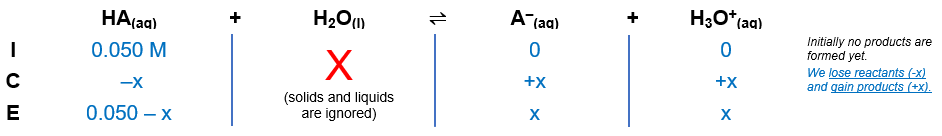# Problem: In a 0.050 M solution of a weak monoprotic acid, [H+] = 1.8 x 10-3. What is its Ka?A. 3.6 x 10-2B. 9.0 x 10-5C. 6.7 x 10-5D. 1.6 x 10-7

###### FREE Expert Solution

Remember that weak acids partially dissociate in water and that acids donate H+ to the base (water in this case).

The dissociation of a weak acid, HA is as follows:

HA(aq) + H2O(l)  H3O+(aq) + A(aq)

From this, we can construct an ICE table. Remember that liquids are ignored in the ICE table and Ka expression.

Let X = initial concentration of HAThe Ka expression for HA is:

$\overline{){{\mathbf{K}}}_{{\mathbf{a}}}{\mathbf{=}}\frac{\mathbf{products}}{\mathbf{reactants}}{\mathbf{=}}\frac{\mathbf{\left[}{\mathbf{H}}_{\mathbf{3}}{\mathbf{O}}^{\mathbf{+}}\mathbf{\right]}\mathbf{\left[}{\mathbf{A}}^{\mathbf{-}}\mathbf{\right]}}{\mathbf{\left[}\mathbf{HA}\mathbf{\right]}}}$

Note that each concentration is raised by the stoichiometric coefficient: [HA], [H3O+] and [A] are raised to 1.

[H3O+] = [A] = x = 1.8 x 10-3

We can then plug this into the Ka expression:

81% (450 ratings)###### Problem Details

In a 0.050 M solution of a weak monoprotic acid, [H+] = 1.8 x 10-3. What is its Ka?

A. 3.6 x 10-2

B. 9.0 x 10-5

C. 6.7 x 10-5

D. 1.6 x 10-7